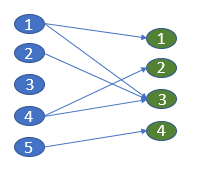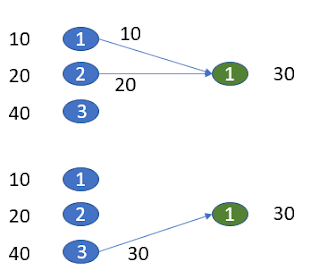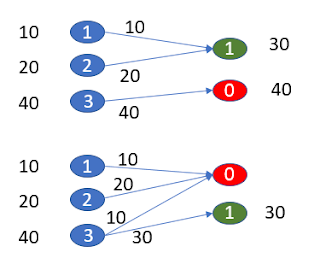## Wednesday, January 30, 2019

### A variant of the transportation problem

In  a problem is described:

• We have $$n$$ groups of volunteers. Groups have different sizes (number of volunteers).
• There are $$m$$ volunteer sites with a certain demand for volunteers.
• Try to assign volunteers to sites such that we don't split up groups if not needed.
As is often the case, a verbal description of the problem gives rise to imprecision. Building a model helps us to surface those issues: it forces us to get the details right and have some consistency.

We can recognize a transportation model in the description of the problem. We have $$n$$ supply nodes (the volunteer groups) and $$m$$ demand nodes (the volunteer sites).

Transportation model
\begin{align} \min & \sum_{i,j} \color{DarkBlue} c_{i,j} \color{DarkRed} x_{i,j}\\ & \sum_j \color{DarkRed} x_{i,j} \le \color{DarkBlue}{\mathit{Supply}}_{i} &&\forall i \\ & \sum_i \color{DarkRed} x_{i,j} = \color{DarkBlue}{\mathit{Demand}}_{j} &&\forall j \\ & \color{DarkRed}x_{i,j} \ge 0 \end{align}

Here we assume total supply is equal or exceeds total demand. If total demand ls larger than total supply, we can use as constraints \begin{align}& \sum_j \color{DarkRed} x_{i,j} = \color{DarkBlue}{\mathit{Supply}}_{i} &&\forall i \\ & \sum_i \color{DarkRed} x_{i,j} \le \color{DarkBlue}{\mathit{Demand}}_{j} &&\forall j \end{align} In the special case where total demand is equal to total supply, we can use either formulation, or alternatively  \begin{align} & \sum_j \color{DarkRed} x_{i,j} = \color{DarkBlue}{\mathit{Supply}}_{i} &&\forall i \\ & \sum_i \color{DarkRed} x_{i,j} = \color{DarkBlue}{\mathit{Demand}}_{j} &&\forall j \end{align}

OK, we had to make one assumption. Besides that we have two more issues:

• The variables $$x_{i,j}$$ should be integer value: we cannot divide up a volunteer.
• The objective is not correct. We need somehow to model "split up as few groups as possible".  One interpretation could be: minimize the number of links $$i \rightarrow j$$ that we use. Putting it differently: find the sparsest solution for $$x_{i,j}$$.Bipartite graph representation
A model that minimizes the number of used links is:

\begin{align} \min & \sum_{i,j} \color{DarkRed} y_{i,j}\\ & \sum_j \color{DarkRed} x_{i,j} \le \color{DarkBlue}{\mathit{Supply}}_{i} &&\forall i \\ & \sum_i \color{DarkRed} x_{i,j} = \color{DarkBlue}{\mathit{Demand}}_{j} &&\forall j \\ & \color{DarkRed}x_{i,j} \le \color{DarkBlue}x^{\color{DarkBlue}up}_{i,j} \cdot \color{DarkRed} y_{i,j} \\ & \color{DarkRed}x_{i,j} \in \{0,1,\dots, \color{DarkBlue}x^{\color{DarkBlue} {up}}_{i,j}\} \\ & \color{DarkRed}y_{i,j} \in \{ 0, 1 \} \\ & \color{DarkBlue} x^{\color{DarkBlue} {up}}_{i,j} = \min(\color{DarkBlue}{\mathit{Supply}}_{i},\color{DarkBlue}{\mathit{Demand}}_{j}) \end{align}

Let's test this with some random data:

----     18 PARAMETER size  volunteers in group

group1   47,    group2  212,    group3  140,    group4   79,    group5   76,    group6   60,    group7   91
group8  215,    group9   21,    group10 128,    group11 250,    group12 147

----     18 PARAMETER request  needed by site

site1 397,    site2 306,    site3  55,    site4 257,    site5  66

----     18 PARAMETER numvolunteer         =         1466  total volunteers
PARAMETER numrequest           =         1081  total requests


The solution can look like:

----     43 VARIABLE y.L  link used

site1       site2       site3       site4       site5

group2                        1
group3                                                1
group4                                                            1
group5                                    1
group8                        1
group10                                               1
group11           1
group12           1

----     43 VARIABLE x.L  flow

site1       site2       site3       site4       site5

group2                       91
group3                                              140
group4                                                           66
group5                                   55
group8                      215
group10                                             117
group11         250
group12         147

----     43 VARIABLE z.L                   =        8.000  objective


There are specialized algorithms for the transportation model. I often use LP solvers for this, as they provide a little bit more flexible and have very competitive performance. I don't think there is an algorithm for the "min number of links" problem. A MIP formulation seems appropriate.Is minimizing number of links the best approach?

A disadvantage of my approach is that using a partial group has the same cost as using a complete group. The picture above illustrates the issue. Arguably one link related to incomplete use of a group may be not as good as two links but using up all supply. Of course if we have a balanced problem, i.e. total supply = total demand, there is no issue with this. In that case all groups will be used completely, so the second case (one link, but leaving leftover supply) will not happen. The question remains: what would be a better approach for unbalanced problems?

Well, one interesting fix is: add an extra dummy node that handles leftover demand or supply. In our case supple exceeds demand so we add an extra demand node. Its demand is previous total demand $-$ total supply. The picture becomes:First assignment is better: 3 active links vs 4

When we do this for our original data set we see:

----     47 VARIABLE y.L  link used

site1       site2       site3       site4       site5       dummy

group1                                                1
group2                        1           1
group3                                                1           1
group4                                                                        1
group5                                                1
group6                                                            1
group7                                                                        1
group8                                                                        1
group9                        1
group10                       1
group11           1
group12           1

----     47 VARIABLE x.L  flow

site1       site2       site3       site4       site5       dummy

group1                                               47
group2                      157          55
group3                                              134           6
group4                                                                       79
group5                                               76
group6                                                           60
group7                                                                       91
group8                                                                      215
group9                       21
group10                     128
group11         250
group12         147

----     47 VARIABLE z.L                   =       14.000  objective


This now solved a balanced problem, and more accurately counts if groups are split up. The optimization model is the same, just the data has been updated with a dummy demand node.

#### References

1. Algorithm for optimally matching certain sized volunteer groups with volunteer sites asking for specific numbers of volunteers?, https://stackoverflow.com/questions/54371450/algorithm-for-optimally-matching-certain-sized-volunteer-groups-with-volunteer-s